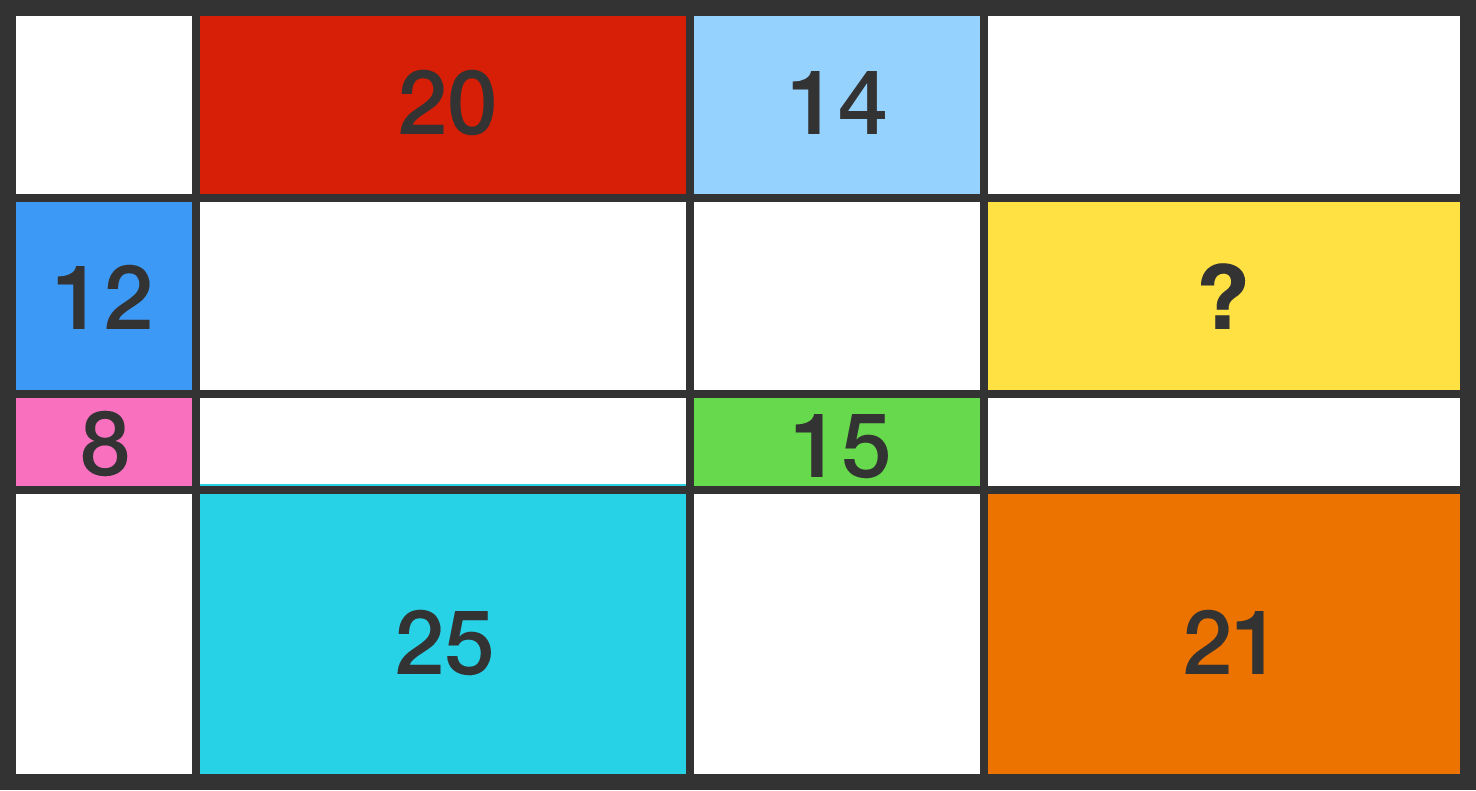# Rectangles in rectangle

Geometry Level 3

A rectangle is divided into 16 smaller rectangles, the areas of seven of which are known to us by the numbers in the figure (which is not drawn to scale).What is the area of the yellow rectangle?

×